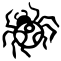## Scatter pictures with Google Charts

In a recent post on his blog Matt Cutts asks:

I almost wanted to call this post “Stupid Google Tricks” :-) What fun diagrams can you imagine making with the Google Charts Service?

Here’s a stupid trick: you can use the Python Imaging Library to convert a picture into a URL which Google charts will render as the original picture.

Here’s the original picture:here’s the version served up by Google charts:here’s the code:

```import Image
import string

def scatter_pixels(img_file):
"""Return the URL of a scatter plot of the supplied image

The image will be rendered square and black on white. Adapt the
code if you want something else.
"""
# Use simple chart encoding. To make things really simple
# use a square image where each X or Y position corresponds
# to a single encode value.
simple = string.uppercase + string.lowercase + string.digits
rsimple = simple[::-1] # Google charts Y reverses PIL Y
w = len(simple)
W = w * 3
img = Image.open(img_file).resize((w, w)).convert("1")
black_pels = [(x, y) for x in range(w) for y in range(w)
if pels[x, y] == 0]
xs = "".join(simple[x] for x, _ in black_pels)
ys = "".join(rsimple[y] for _, y in black_pels)
sqside = 3.0
return (
"cht=s&"                          # Draw a scatter graph
"chd=s:%(xs)s,%(ys)s&"            # using simple encoding and
"chm=s,000000,1,2.0,%(sqside)r,0&"# square black markers
"chs=%(W)rx%(W)r"                 # at this size.
) % locals()

```

and here’s the url it generates: